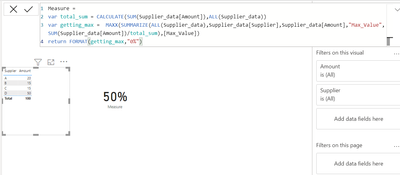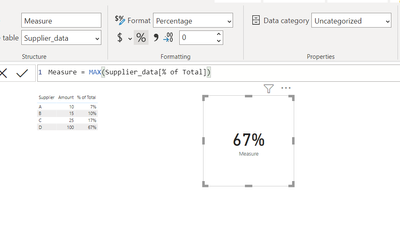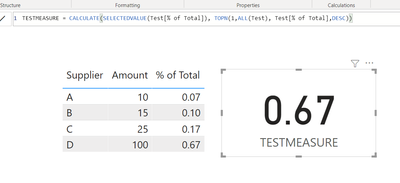cancel
Showing results for
Did you mean:

Fabric is Generally Available. Browse Fabric Presentations. Work towards your Fabric certification with the Cloud Skills Challenge.Helper II

## Need a Dax to return % of top product

Hello,

I am putting together some dynamic text and need a Dax that will return the % that the top supplier makes up of total.

In the example below, I would want DAX to return 50%.

I need to show is 50% i.e. (25+25)/100 in the example below since supplier D is the highest of them all.
 Supplier Amount A 20 B 15 C 15 D 25 D 25 Total 100

I do have following measure in my report if that helps:

Actual Amount = ROUND(SUM('Transactions'[Amount]),2)

2 ACCEPTED SOLUTIONSCommunity Champion

You can create a measure with below code

``````Measure =
var total_sum = CALCULATE(SUM(Supplier_data[Amount]),ALL(Supplier_data))
var getting_max =  MAXX(SUMMARIZE(ALL(Supplier_data),Supplier_data[Supplier],Supplier_data[Amount],"Max_Value",SUM(Supplier_data[Amount])/total_sum),[Max_Value])
return FORMAT(getting_max,"0%")``````Best Regards,
Samarth

If this post helps, please consider accepting it as the solution to help the other members find it more quickly.Helper II

This was helpful. I had to tweak the formula a little. Here was the final formula:

``````% of highest Supplier =
var total_supplier = CALCULATE([Actual Amount],'Find Suppliers - Addl Info'[Supplier]<>BLANK())
var getting_max =  MAXX(SUMMARIZE(FILTER('Fact Table','Fact Table'[Supplier ID]<>BLANK()),'Find Suppliers - Addl Info'[Supplier],"Max_Value",SUM('Fact Table'[GL Amount])/total_supplier),[Max_Value])
return

FORMAT(getting_max,"0.0%")``````

6 REPLIES 6Community Champion

Hi @zzakir ,

If i am taking it correctly you just need a highest % of total. You can take a Max of "% of total column" column.

``Measure = MAX(Supplier_data[% of Total])``Please let me know if this is the output you looking.

Best Regards,
Samarth

If this post helps, please consider accepting it as the solution to help the other members find it more quickly.Helper II

@Samarth_18 I have editted my original question to be clearer.

I do not have % column.Community Champion

You can create a measure with below code

``````Measure =
var total_sum = CALCULATE(SUM(Supplier_data[Amount]),ALL(Supplier_data))
var getting_max =  MAXX(SUMMARIZE(ALL(Supplier_data),Supplier_data[Supplier],Supplier_data[Amount],"Max_Value",SUM(Supplier_data[Amount])/total_sum),[Max_Value])
return FORMAT(getting_max,"0%")``````Best Regards,
Samarth

If this post helps, please consider accepting it as the solution to help the other members find it more quickly.Helper II

This was helpful. I had to tweak the formula a little. Here was the final formula:

``````% of highest Supplier =
var total_supplier = CALCULATE([Actual Amount],'Find Suppliers - Addl Info'[Supplier]<>BLANK())
var getting_max =  MAXX(SUMMARIZE(FILTER('Fact Table','Fact Table'[Supplier ID]<>BLANK()),'Find Suppliers - Addl Info'[Supplier],"Max_Value",SUM('Fact Table'[GL Amount])/total_supplier),[Max_Value])
return

FORMAT(getting_max,"0.0%")``````Solution Sage

Hi zzakir,

Is this the result you're looking for? It takes the % value of the top 1 supplier based on the [% of total] column.

``TESTMEASURE = CALCULATE(SELECTEDVALUE(Test[% of Total]), TOPN(1,ALL(Test), Test[% of Total],DESC))``Best regards,

Tim

Proud to be a Super User!Helper II

Hello @timg,
This is close to what I want. Perhaps I should've asked my original question differently:

I have a transaction table below.

I need to show is 50% i.e. (25+25)/100 in the example below in a card like you had 67% above.
 Supplier Amount A 20 B 15 C 15 D 25 D 25 Total 100

I do have following measure in my report if that helps:

Actual Amount = ROUND(SUM('Transactions'[Amount]),2)Announcements#### Power BI Monthly Update - November 2023

Check out the November 2023 Power BI update to learn about new features.#### Fabric Community News unified experience

Read the latest Fabric Community announcements, including updates on Power BI, Synapse, Data Factory and Data Activator.#### The largest Power BI and Fabric virtual conference

130+ sessions, 130+ speakers, Product managers, MVPs, and experts. All about Power BI and Fabric. Attend online or watch the recordings.Top Solution Authors
Top Kudoed Authors
Users online (1,571)